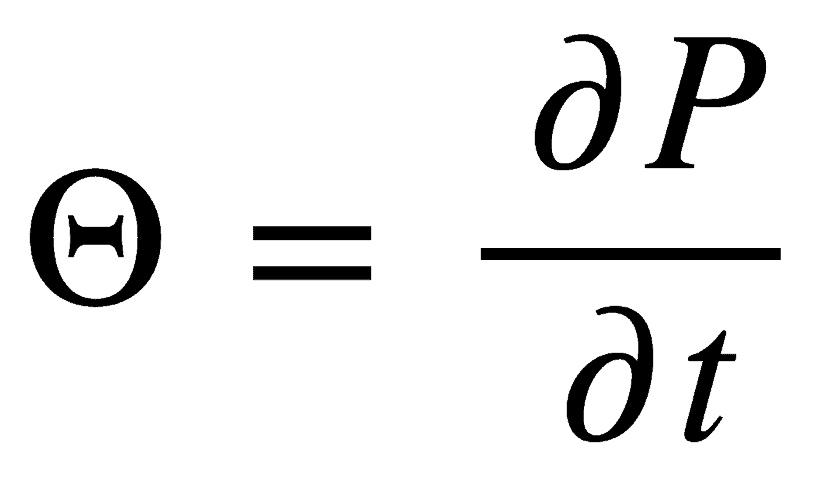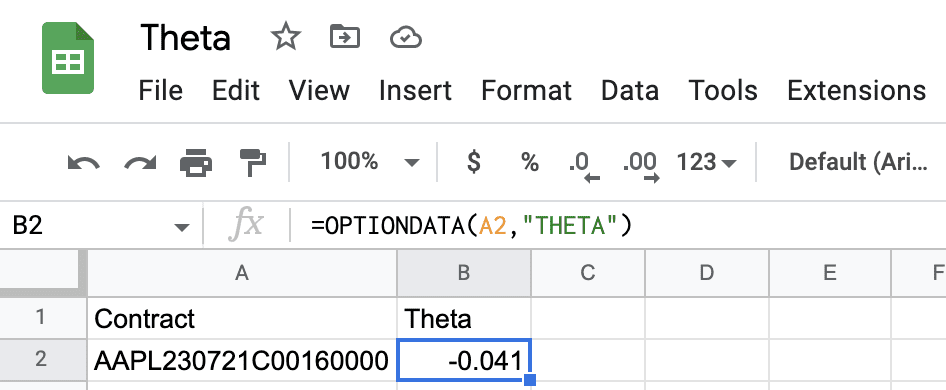February 14, 2023

# How to Calculate Theta For An OptionWhen buying or selling an option, one of the most important options greeks is theta, which also referred to as time decay. Most broker platforms will calculate the value of theta automatically for you. If you calculate theta for yourself using your spreadsheet, be aware that the theta formula is yearly. Most option traders find it more useful to convert the value to a daily number and understand the daily time decay for the option they are considering. That’s why broker platforms will deliver theta as a daily value to users.

## Calculating Theta Using Black Scholes

The yearly theta value is calculated by dividing the rate of change in the option premium paid by the rate of change in time. This value should then be divided by 252 to convert the annual value into a value for a single trading day or by 365 to convert the value into calendar days.

##Calculate Theta With Market Data’s Add-on
Let’s see an example using the \$160 AAPL Jul 21, 2023 Call. The option symbol for this call is AAPL230721C00160000. After installing the Add-on, use the formula `=OPTIONDATA("AAPL230721C00160000","theta")`.  As you can see, that’s much easier than trying to calculate theta manually using Black Scholes.